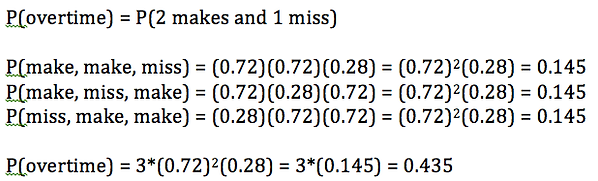top of page

## Chapter 6 - Day 6 - Lesson 6.3

##### Learning Targets
• Determine whether the conditions for a binomial setting are met.

• Calculate and interpret probabilities involving binomial distributions.

##### Activity: Is it Smart to Foul at the End of the Game?

This activity is built around an incredible lesson available at Mathalicious (this one is free!). While you’re at it, sign up for a trial! We’ve been members for years.  They have really high quality engaging lessons!We scaffolded students through probability calculations as we slowly revealed the outcome of each free throw. This lesson has some great connections to Chapter 5 (independent events, conditional probability), but our focus was on developing the conceptual understanding of the binomial formula.  Instead of simply giving them the binomial formula, this lesson helps students to understand the components of the binomial formula (experience first, formalize later!).

The best context for discussing the binomial formula is when calculating the probability of overtime.So what does the 3 represent?  It is the number of ways of making 2 and missing 1.  This can also be calculated using a combination (3 choose 2).  Discussing this idea with students is vital if they are to understand the binomial formula.

bottom of page